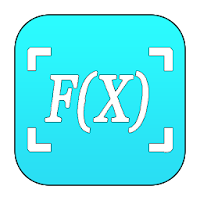Photo Calculator - Smart Calculator Math Solver# Photo Calculator - Smart Calculator Math Solver

## Solve math problems and show math answers with smart calculator math scanner

Developer: Florahugs
App Size: 4.4M
Release Date: Sep 14, 2019
Price: Free
3.9
11 Ratings
Size
4.4M

### Screenshots for App

Mobile
Use your phone's camera to solve equations with Smart Calculator - Math Calculator & Math Solver.

Are you looking for quick and accurate math answers but not specific math calculator? With Photo Calculator, your phone can become Math scanner to solve math problem and immediately receive the photo calculator answers.

Smart Calculator can simply snap photo calculator and math solver instantly delivers the photo calculator answers, math solution while also providing step by step solver.

Math Solver is a valuable tool for a parent helping with his kid 's math homework and attempting to remember middle-school algebra, photo calculator.

Math Graph – you draw graph.

✦Math app can help students check their work and show them how to arrive at the correct math answers. And Smart Calculator - Math solver app can help parents brush up on math lessons learned long ago.✦

✍ FEATURES OF SMART CALCULATOR ✍
ღ Photo Calculator app calculates mathematics problems with camera.
ღ Click a photo calculator, crop the portion and save it.
ღ Math Problem Solver can solve simple as well as complex math graph problems.
ღ Smart Calculator also contains manual math calculator.
ღ Scientific Calculator - Camera calculator simple to grasp and easy to use the usual arithmetic functions and exponentiation.
ღ Math Graph in polar coordinates.
ღ Math Graph parametric solve equations.
ღ Math Scanner with no internet required.
ღ Step by step solutions for different methods to solve math graph problem.
ღ Math Solver - Camera calculator with free graphing calculator and Tables, algebra calculator or math calculator.
ღ Math Calculator can solve simple to advanced math questions.
ღ Smart Calculator instants math solutions from algebra to trig and calculus.

Students will certainly use Math Calculator to do the heavy lifting for them. Math scanner can help their homework with integral math app, graphing calculator, math calculator, photo calculator and math problem solver math solution.

☝MAIN FUNTION of SMART CALCULATOR☝
★ Basic Calculator : Basic mathematics free functions with percentage.
★ Photo Calculator : Good math homework helper which solves math problems by taking photo.
★ Smart Calculator : Based on casio 570 with math solution
★ Equation calculator : Solve your math homework efficiently.
★ Graphing calculator : Easily math graph functions, solve equations, find special points of functions, save and share your results

Photo calculator - Camera calculator
You don’t need to input all the exercises one by one but take a picture and then you can get the solutions at once with free graphing calculator.

Basic calculator
The basic photo calculator in this Photo Calculator app contains add up, subtraction and division. Smart Calculator includes the percentage calculation and brackets.

Smart calculator
Slide up from the bottom of the keyboard and the scientific calculator will be shown. This all-in-one smart calculator, math app contains all the scientific calculations based on Casio 570.

Graphing calculator
Active analysis of functions, for better free graphing calculator of 2D functions with singularity points. Plot polar, cartesian, or math graph,mathematics apps

Equation calculator
With smart calculator, you just need to enter the formula and the equation calc will give you answers automatically.

? Graphing Calculator by Math graph is a scientific graphing calculator integrated with algebra and is an smart calculator tool for all students, or just anyone who needs more than what a basic calculator offers.
? Scientific calculator is designed to replace bulky and costly handheld free graphing calculator and works on virtually any Android phone or tablet,mathematics apps

Smart Calculator - Math Calculator & Math Solver is an easy to use application for all students.Smart Calculator app is 100% FREE!